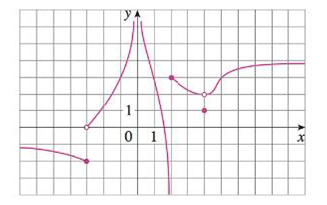Chapter 2, Problem 1RE

Chapter
Section
Textbook Problem

The graph of f is given.(a) Find each limit, or explain why it does not exist.(i) lim x → 2 + f ( x ) (ii) lim x → − 3 + f ( x ) (iii) lim x → − 3 f ( x ) (iv) lim x → 4 f ( x ) (v) lim x → 0 f ( x ) (vi) lim x → 2 − f ( x ) (vii) lim x → ∞ f ( x ) (viii) lim x → − ∞ f ( x ) (b) State the equation of the horizontal asymptotes.(c) State the equations of the vertical asymptotes.(d) At what numbers is f discontinuous? Explain.

(a)

To determine

To find: The limit of each of the given functions and explain if the limit does not exist.

Explanation

Calculation:

Section (i)

Obtain the value of limx2+f(x).

From the given graph, it is found that the curve move towards y=3 as x approaches 2 from the right, that is x>2.

Therefore, limx2+f(x)=3.

Section (ii)

Obtain the value of limx3+f(x).

From the given graph, it is found that the curve move towards y=0 as x approaches 3 from the right, that is x>3.

Therefore, limx3+f(x)=0.

Section (iii)

Obtain the value of limx3f(x).

From section (ii),

limx3+f(x)=0

From the given graph, it is found that the curve move towards y=2 as x approaches 3 from the left, that is x<3.

Thus, limx3+f(x)=2

Recall the definition that limxaf(x) exists only when limxaf(x)=limxa+f(x).

Since limx3+f(x)limx3f(x)

Therefore, limx3f(x) does not exists

(b)

To determine

To state: The equation of horizontal asymptotes.

(c)

To determine

To state: The equation of vertical asymptotes.

(d)

To determine

To find: The numbers at which f is discontinuous.

Still sussing out bartleby?

Check out a sample textbook solution.

See a sample solution

The Solution to Your Study Problems

Bartleby provides explanations to thousands of textbook problems written by our experts, many with advanced degrees!

Get Started

Simplify the expressions in Exercises 97106. x3/2x5/2

Finite Mathematics and Applied Calculus (MindTap Course List)

Convert the following mixed numbers to improper fractions. 14.

Contemporary Mathematics for Business & Consumers

CHECKPOINT Suppose that Find

Mathematical Applications for the Management, Life, and Social Sciences